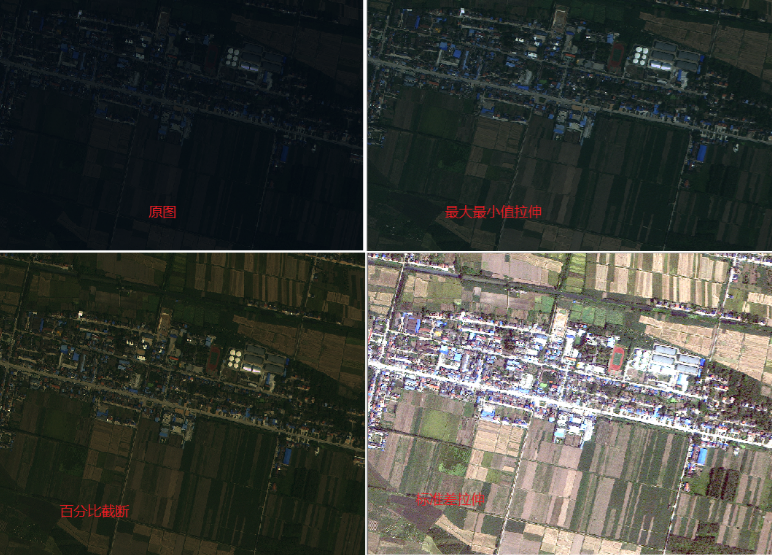2018-05-03 16:14:13 LEILEI18A 阅读数 4374
• ###### 数据结构基础系列(2)：线性表

数据结构课程是计算机类专业的专业基础课程，在IT人才培养中，起着重要的作用。课程按照大学计算机类专业课程大纲的要求，安排教学内容，满足需要系统学习数据结构的人。系列课程包含11个部分，本课为第2部分，线性表的逻辑结构，线性表的顺序表和链表两种存储结构，以及在各种存储结构中基本运算的实现，还通过相关的应用案例介绍了相关知识的应用方法。

19313 人正在学习 去看看 贺利坚

1）直接线性拉伸：（直接归一化，然后放缩到指定大小范围）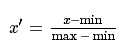import cv2

import numpy as np

gray_new=( gray-gray.min() ) / ( gray.max()-gray.min() ) # 归一化到0-1

maxout=255

minout=0

gray_out=gray_new * （maxout -minout） # maxout=255   minout=0

gray_out=np.uint8( gray_out )

2）裁剪线性拉伸：（去掉2%百分位以下的数，去掉98%百分位以上的数，上下百分位数一般相同，并设置输出上下限）

import cv2
import numpy as np

d2=np.percentile( gray,2 )
u98=np.percentile( gray,98 )

maxout=255
minout=0

gray_new=minout + ( (gray-d2) / (u98-d2) ) * (maxout - minout)
gray_new[gray_new < minout]=minout
gray_new[gray_new > maxout]=maxout

gray_out=np.uint8(gray_new)

3）分段式 线性拉伸（百度图片）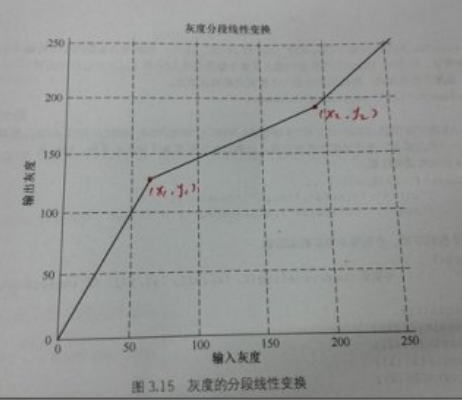####################################################

envi 中对应的线性拉伸实现：

ENVI对打开的一幅遥感影像默认是2%的线性拉伸，当然只是显示效果发生变化，亮度得到提升，但是像元值并没有发生改变；这里的Linear2%是指将直方图累积在2%至98%之间的像元值拉伸，取直方图累积在2%处对应的光谱值为MinValue，98%处对应的光谱值为MaxValue，那么可解释为如果像元值大于MinValue且小于MaxValue，则将其拉伸至0-255；如果像元值小于MinValue，那么将其改为MinValue；如果像元值大于MaxValue，那么将其改为255。

####################################################

2019-07-16 13:03:03 wujuxKkoolerter 阅读数 271
• ###### 数据结构基础系列(2)：线性表

数据结构课程是计算机类专业的专业基础课程，在IT人才培养中，起着重要的作用。课程按照大学计算机类专业课程大纲的要求，安排教学内容，满足需要系统学习数据结构的人。系列课程包含11个部分，本课为第2部分，线性表的逻辑结构，线性表的顺序表和链表两种存储结构，以及在各种存储结构中基本运算的实现，还通过相关的应用案例介绍了相关知识的应用方法。

19313 人正在学习 去看看 贺利坚

# 图像灰度线性变换

$g(x,y) = \frac{d - c}{b - a}[f(x,y) - a] + c \tag{9-1}$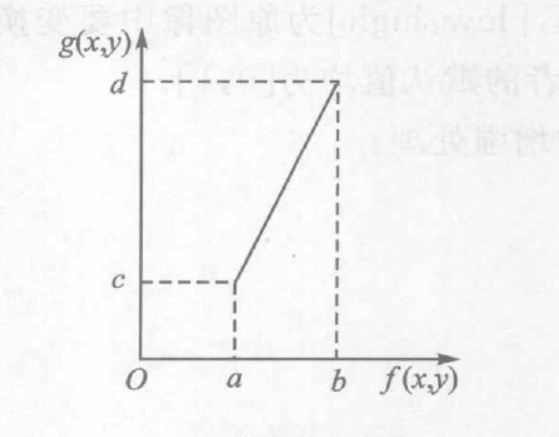$g(x,y) = \begin{cases} c & 0 \leq f(x,y) \leq a \\ \frac{d-c}{b-a}[f(x,y) - a] + c & a \lt f(x,y) \lt b \\ d &b \lt f(x,y) \leq M \end{cases} \tag{9-2}$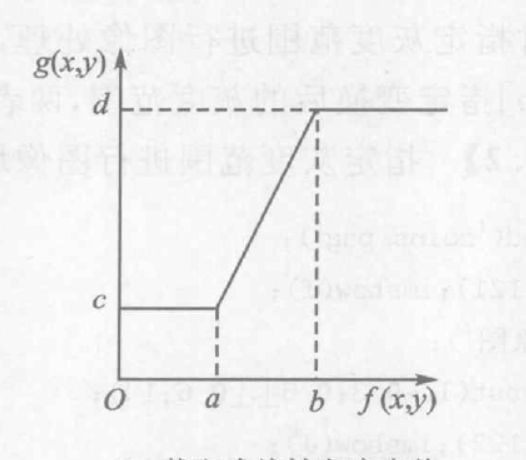Python实现代码如下：

def linear_transform(src,c = 0,low=0,high=1,bottom=0,up=1):
assert high >= low and high >= 0 and high <= 1 and low >= 0 and low <= 1
assert up > bottom and up >= 0 and up <= 1 and bottom >= 0 and bottom <= 1

dst = np.zeros_like(src).astype(np.float32)
dst = (up - bottom) / (high - low) * (src.astype(np.float32)-low) + c
dst = np.clip(dst,0,255).astype(np.uint8)
return dst2017-03-16 09:21:22 muyuyi_1999 阅读数 2029
• ###### 数据结构基础系列(2)：线性表

数据结构课程是计算机类专业的专业基础课程，在IT人才培养中，起着重要的作用。课程按照大学计算机类专业课程大纲的要求，安排教学内容，满足需要系统学习数据结构的人。系列课程包含11个部分，本课为第2部分，线性表的逻辑结构，线性表的顺序表和链表两种存储结构，以及在各种存储结构中基本运算的实现，还通过相关的应用案例介绍了相关知识的应用方法。

19313 人正在学习 去看看 贺利坚

#include <opencv2/core/core.hpp>
#include <opencv2/highgui/highgui.hpp>
#include <iostream>

using namespace std;
using namespace cv;

Mat XianChange(Mat &img, double alt, int base)//灰度线性变化、
{
CV_Assert(img.depth() != sizeof(uchar));  //声明只对深度8bit的图像操作
Mat temp = img.clone();

int channels = img.channels();            //获取图像channel
int nrows = img.rows;                     //矩阵的行数
int ncols = img.cols*channels;             //矩阵的总列数=列数*channel分量数
if (img.isContinuous())               //判断矩阵是否连续，若连续，我们相当于只需要遍历一个一维数组
{
ncols *= nrows;
nrows = 1;                 //一维数组
}
//遍历像素点灰度值
for (int i = 0; i<nrows; i++)
{
uchar *p = temp.ptr<uchar>(i);    //获取行地址
for (int j = 0; j<ncols; j++)
{
p[j] = alt*p[j] + base;//修改灰度值

if (p[j] > 255)
p[j] = 255;
if (p[j] < 0)
p[j] = 0;
}
}
return temp;
}

Mat XianStretch(Mat &img, int a, int b, int c, int d)//线性灰度拉伸，将[a,b]拉伸到[c,d]
{
CV_Assert(img.depth() != sizeof(uchar));  //声明只对深度8bit的图像操作
Mat temp = img.clone();

int channels = img.channels();            //获取图像channel
int nrows = img.rows;                     //矩阵的行数
int ncols = img.cols*channels;             //矩阵的总列数=列数*channel分量数
if (img.isContinuous())               //判断矩阵是否连续，若连续，我们相当于只需要遍历一个一维数组
{
ncols *= nrows;
nrows = 1;                 //一维数组
}
//遍历像素点灰度值
for (int i = 0; i<nrows; i++)
{
uchar *p = temp.ptr<uchar>(i);    //获取行地址
for (int j = 0; j<ncols; j++)
{
if (p[j] < a)
p[j] = c / b * p[j];
else if (p[j] > a && p[j] < b)
p[j] = (d - c) / (b - a) * (p[j] - a) + c;
else
p[j] = (255 - d) / (255 - b) *(p[j] - b) + d;
}
}
return temp;
}

Mat HistogramEqu(Mat &img)               //直方均衡
{
CV_Assert(img.depth() != sizeof(uchar));  //声明只对深度8bit的图像操作
Mat temp = img.clone();
int grayNum = { 0 };
int grayMap = { 0 };

int channels = img.channels();            //获取图像channel
int nrows = img.rows;                     //矩阵的行数
int ncols = img.cols*channels;             //矩阵的总列数=列数*channel分量数
int allPixel = nrows*ncols;               //图像的像素总数
int c;                                    //用于计算累积分布概率

if (img.isContinuous())               //判断矩阵是否连续，若连续，我们相当于只需要遍历一个一维数组
{
ncols *= nrows;
nrows = 1;    //一维数组
}
//遍历像素点灰度值
for (int i = 0; i<nrows; i++)
{
uchar *p = temp.ptr<uchar>(i);    //获取行地址
for (int j = 0; j<ncols; j++)
{
grayNum[p[j]]++;        //原图像的直方图
}
}

for (int i = 0; i < 256; i++)     //重新定义新的直方图
{
c = 0;
for (int j = 0; j<=i; j++)
{
c += grayNum[j];
grayMap[i] = int (255 * c / allPixel);

}
//printf("%d %d\n", i, grayMap[i]);
}

for (int i = 0; i < nrows; i++)     //重新定义新的直方图
{
uchar *p = temp.ptr<uchar>(i);    //获取行地址
for (int j = 0; j < ncols; j++)
{
p[j] = grayMap[p[j]];
}
}
return temp;
}

int main()
{
string picName = "lena256.jpg";

imshow("变换前", A);

Mat B = XianChange(A, 1, 20);//根据需要设置不同的参数
imshow("线性变换后", B);

Mat C = XianStretch(A, 10, 100, 20, 50);//根据需要设置不同的参数
imshow("线性拉伸后", C);

Mat D = HistogramEqu(A);
imshow("直方均衡后", D);

waitKey();
return 0;
}2014-12-10 22:57:57 whustyle 阅读数 1569
• ###### 数据结构基础系列(2)：线性表

数据结构课程是计算机类专业的专业基础课程，在IT人才培养中，起着重要的作用。课程按照大学计算机类专业课程大纲的要求，安排教学内容，满足需要系统学习数据结构的人。系列课程包含11个部分，本课为第2部分，线性表的逻辑结构，线性表的顺序表和链表两种存储结构，以及在各种存储结构中基本运算的实现，还通过相关的应用案例介绍了相关知识的应用方法。

19313 人正在学习 去看看 贺利坚

承接上一篇博客，接下来实现以下灰度图像的线性拉伸变换，具体理论就不赘述了，学过数字图像处理的基本都知道，下面来看看如何通过VC6.0的MFC编程实现它。void CImageProcessView::OnLinertrans()
{

}

下面，我们需要创建一个对话框，来接受用户设置的线性变换参数，最小值和最大值，可以右键Dialog，InsertDialog，设置对话框ID为：IDD_DIALOG_LinerTransSet为对话框创建类，双击对话框，提示创建新的类，选择确定，设置类参数如下：这些准备工作做好了之后，便可以实现前面空着的OnLinertrans函数了，先在View.cpp文件上方添加头文件包含# include "LinerTransDlg.h"，然后实现OnLinertrans，

void CImageProcessView::OnLinertrans()
{
CLinerTransDlg linedlg;

if(linedlg.DoModal()==IDOK)
{
UpdateData(TRUE);
CImageProcessDoc* pDoc=GetDocument();
ASSERT_VALID(pDoc);

BYTE* pData=pDoc->m_bmpFile.m_pImgDat;

int cols=pDoc->m_bmpFile.m_Cols;
int rows=pDoc->m_bmpFile.m_Rows;
int min=linedlg.m_nMin;
int max=linedlg.m_nMax;

float mingray=255,maxgray=0;

for(int r=0;r<rows;r++)
{
for(int c=0;c<cols;c++)
{
if(*(pData+cols*r+c)<mingray)
mingray=*(pData+cols*r+c);

if(*(pData+cols*r+c)>maxgray)
maxgray=*(pData+cols*r+c);

}
}
float a=(min-max)/(mingray-maxgray);
for(r=0;r<rows;r++)
{
for(int c=0;c<cols;c++)
{
int gray=int(min+a*(*(pData+cols*r+c)-mingray));
*(pData+cols*r+c)=BYTE(gray);
}
}
Invalidate();
}
}2018-04-14 20:41:18 chijingjing 阅读数 1989
• ###### 数据结构基础系列(2)：线性表

数据结构课程是计算机类专业的专业基础课程，在IT人才培养中，起着重要的作用。课程按照大学计算机类专业课程大纲的要求，安排教学内容，满足需要系统学习数据结构的人。系列课程包含11个部分，本课为第2部分，线性表的逻辑结构，线性表的顺序表和链表两种存储结构，以及在各种存储结构中基本运算的实现，还通过相关的应用案例介绍了相关知识的应用方法。

19313 人正在学习 去看看 贺利坚

上一篇文章介绍了百分比截断拉伸，本篇介绍标准差拉伸，线性拉伸的思想还是不变，寻找极值范围，极值范围的值直接映射到目标像素极值上，其他值仍旧线性拉伸。

标准差拉伸寻找的极值有一个参数，那就是标准差倍数，ArcGis默认2.5倍的标准差范围作为极值范围，Erdas默认2倍标准差范围，也就是超过这个标准差范围的值将直接映射到目标像素范围的极值上。首先放算法：

template<typename T>
inline int CImageCorrectionDlg::StandardDeviationTension(const T * poSrcData, T * poDstData, int nSizex,
T smin, T smax, T dmin, T dmax,
double StandardDeviation, double avg, int Kn)
{
//double k = (double)(dmax - dmin) / (smax - smin);
//double b = (double)(smax*dmin - smin*dmax) / (smax - smin);

////0~K倍标准差 范围的值做映射其他值做极值
//double kb = K*StandardDeviation;
double ucMax = avg + Kn * StandardDeviation;
double ucMin = avg - Kn * StandardDeviation;

double k = (double)(dmax - dmin) / (ucMax - ucMin);
double b = (double)(ucMax*dmin - ucMin*dmax) / (ucMax - ucMin);

if (ucMin <= 0)
{
ucMin = 0;
}
#pragma omp parallel for
for (int i = 0; i < nSizex; i++)
{
if (poSrcData[i] == 0)
{
poDstData[i] = 0;
continue;
}

//当前点的标准差，
//int sd = (T)poSrcData[i] - (T)avg;
if (poSrcData[i] <= ucMin)
{
poDstData[i] = 0;
continue;
}
else if (poSrcData[i] >= ucMax)
{
poDstData[i] = 255;
continue;
}
//else if (poSrcData[i] >= ucMin && poSrcData[i] <= ucMax)
//{
//	poDstData[i] = double(poSrcData[i] - ucMin) / (double)(ucMax - ucMin) * 255;
//}

int tmp = k*poSrcData[i] + b;

if (tmp <= 0)
{
if (poSrcData[i] == 0)
poDstData[i] = 0;
else
poDstData[i] = 1;//原来不是0的如果直接拉伸0将变为nodata
}
else if (tmp >= 255)
poDstData[i] = 255;
else
poDstData[i] = (T)tmp;
}
return 0;
}